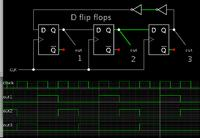# 4 Phases Non-Overlapping Clock Generator

Status
Not open for further replies.

#### a7m3d

##### Newbie level 3
How to implement/design 4 phases non-overlapping clock generator?
I found this figure without enough details about the implementation. I need a clear reference to build the 4 phases non-overlapping clock generator or in general n phases non-overlapping clock generators for example a 3 phases non-overlapping clock generator.The feedback between the 2 flip-flops reserves the state of the output of the 2nd flip-flop. If Q2=0: -> D1=0 -> Q1=0 -> D2=0.
I do not know if I missed something. I will appropriate any help. Thanks.

To get four outputs, add two more flip flops.

It is plain to see why you do not get the same results as my simulation. You must add the NOR gates as shown in my post #2.

The problem is not is the NOR gates, the output of the 4 D flip-flops is the same Q1=Q2=Q3=Q4=0. CLK NOR Q(1 or 2 or 3 or 4) will give the same result which is the CLK complement. I do not know if I missed something. Thank you very much.

The problem is not is the NOR gates, the output of the 4 D flip-flops is the same Q1=Q2=Q3=Q4=0. CLK NOR Q(1 or 2 or 3 or 4) will give the same result which is the CLK complement. I do not know if I missed something. Thank you very much.

Yes, now I am getting a similar result with my simulation. It does not know what initial state to assign the long wire going the length of the circuit.

I tried a few things, attempting to obtain a sensible output. It worked when I put 2 inverters in a row.Simulators are prone to these types of errors.

You see the ways to make like a Johnson Counter sequence .
Synchronous counter decoder, rotating serial shift register.

Unknown Setup/hold times can produce unpredictable results.

But, if you wanted the edges to be non-overlapping as well as each state, then there must be a dead-band between output phases, such as used in full bridge drivers to prevent shoot-thru.

If that were the case, a monopulse or one-shot on the active clock edge can be used to AND or disable the outputs.

Status
Not open for further replies.